# Waveforms

The LIFX LAN protocol supports changing the color of a device over time in accordance with the shape of a waveform. For devices that support multiple zones, these effects will treat all zones as one zone and the entire device will perform the waveform effect as a single color.

These waveforms allow us to combine functions such as fading, pulsing, etc by applying waveform interpolation on the modulation between two colors.

The available waveform values are as follows:

FieldValue
SAW0
SINE1
HALF_SINE2
TRIANGLE3
PULSE4

## Explaining the waveforms

Note that if `transient == true` or the waveform is `SINE` or `TRIANGLE` then the device will return to the original color after the effect. Otherwise the device will not revert to the original color after the effect.

The waveform types can be best explained with the following images:

### SINE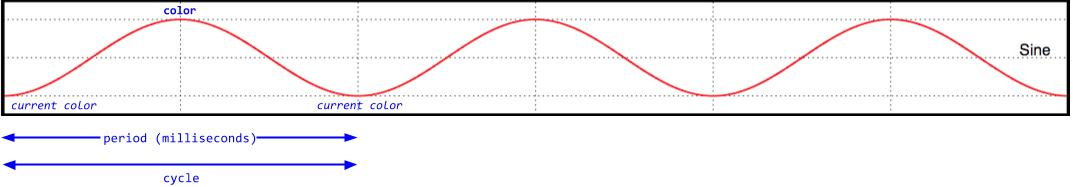• Where `waveform == SINE`, the color will cycle smoothly from current color to `color` and then end back at current color
• The duration of one cycle will last for `period` milliseconds

### PULSE

Pulse waveforms use the `skew_ratio` parameter: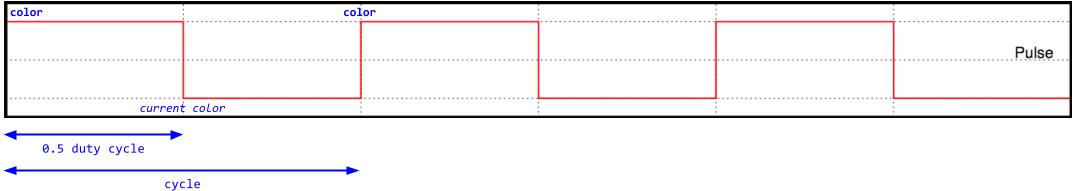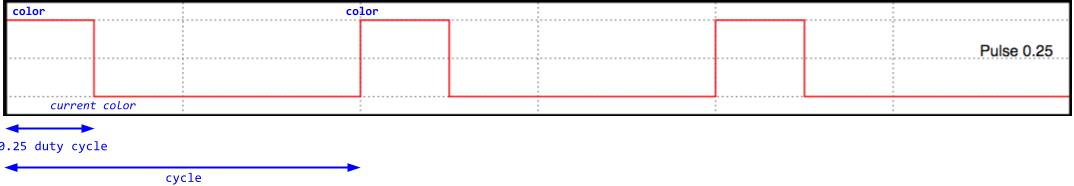Where `waveform == PULSE`, the color will be set immediately to `color`, then to current color after the duty cycle fraction expires

The duty cycle percentage is calculated by applying `1 - skew_ratio` as a percentage of the `cycle` duration and changes the time spent on the original color vs the new color

• `duty_cycle == 0.5`: color will be set to `color` for the first 50% of the cycle period, then to current color until the end of the cycle

• `duty_cycle == 0.25`: color will be set to `color` for the first 25% of the cycle period, then to current color until the end of the cycle

Note that skew_ratio in the message is a signed Int16 that is scaled from 0 to 1.

value in messagescaled skew_ratio
-327680
-163830.25
00.5
163830.75
327671

### Half-sine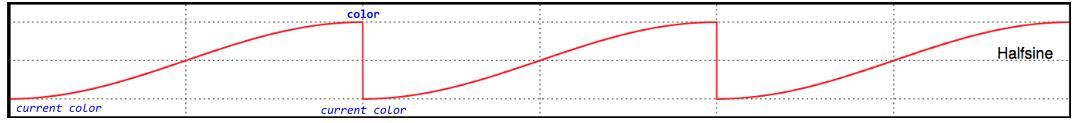• `waveform == HALF_SINE`: light interpolates smoothly from current color to `color`
• Duration of each cycle lasts for `period` milliseconds

### Triangle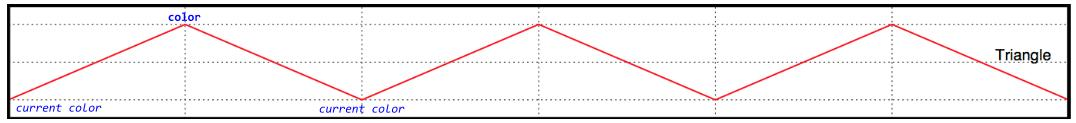• `waveform == TRIANGLE`: light interpolates linearly from current color to `color`, then back to current color
• Duration of each cycle lasts for `period` milliseconds

### Saw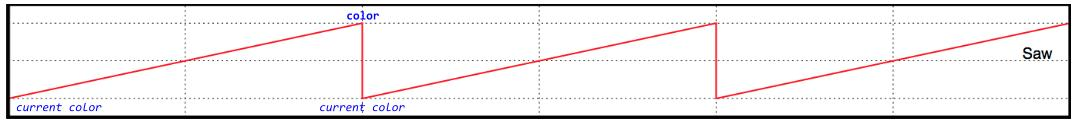• `waveform == Saw`: light interpolates linearly from current color to `color`
• Duration of each cycle lasts for `period` milliseconds